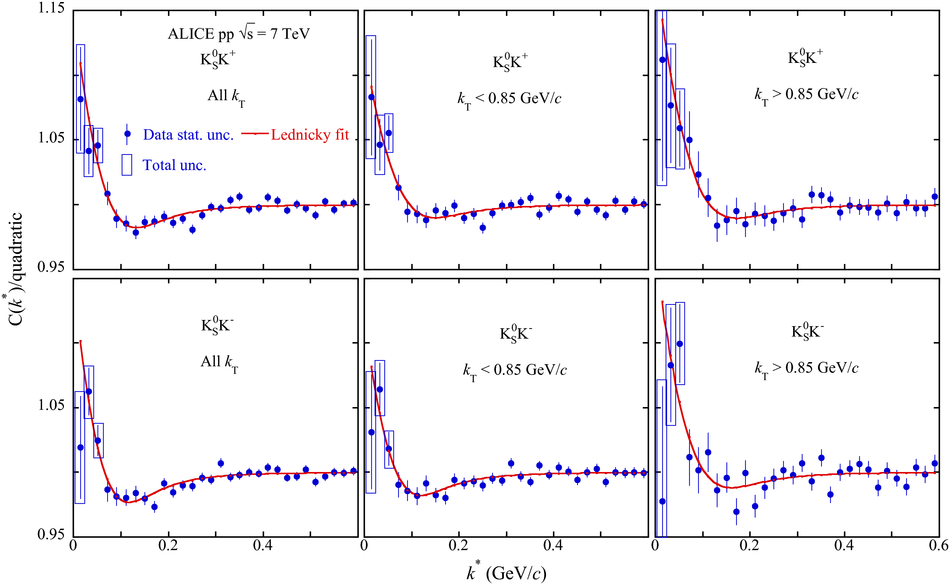# Figure 3

 Correlation functions divided by one of the baseline functions with fits from Eq.~(\ref{eq:corrfit}) for K$^0_{\rm S}$K$^+$ and K$^0_{\rm S}$K$^-$ and $k^*$ fit range (0.0-0.6 GeV/$c$) for the three $k_T$ bins and the quadratic baseline function assumption. Statistical (lines) and the quadratic sum of the statistical and systematic (boxes) uncertainties are shown. For $k^*>0.05$ GeV/$c$, the systematic uncertainties become negligible and the boxes are no longer shown.